# How to Graph a Line Using a Table of Values

How to Graph a Line Using a Table of Values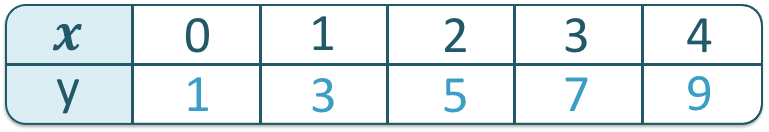• A table of values contains 𝑥 values in ascending order and these are used to calculate y values.
• Substitute each 𝑥 value into the given equation to calculate the y value that goes with it.
• For example, here we have y = 2𝑥 + 1 and we will substitute the values of 𝑥 = 0, 1, 2, 3 and 4.
• The equation tells us to double each 𝑥 value and add 1. We write this number for each 𝑥 value in the corresponding y value row.• Each pair of 𝑥 and y values form a pair of coordinates.
• The 𝑥 coordinate tells us how far right to go and the y coordinate tells us how far up to go.
• Both numbers are required to plot each individual point.

Enter each 𝑥 value into the equation given to work out the y value. Each pair of 𝑥 and y values form a pair of coordinates.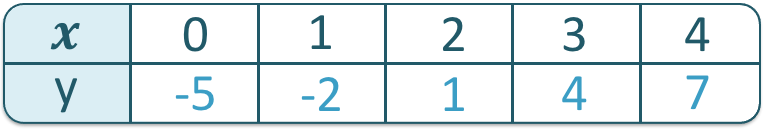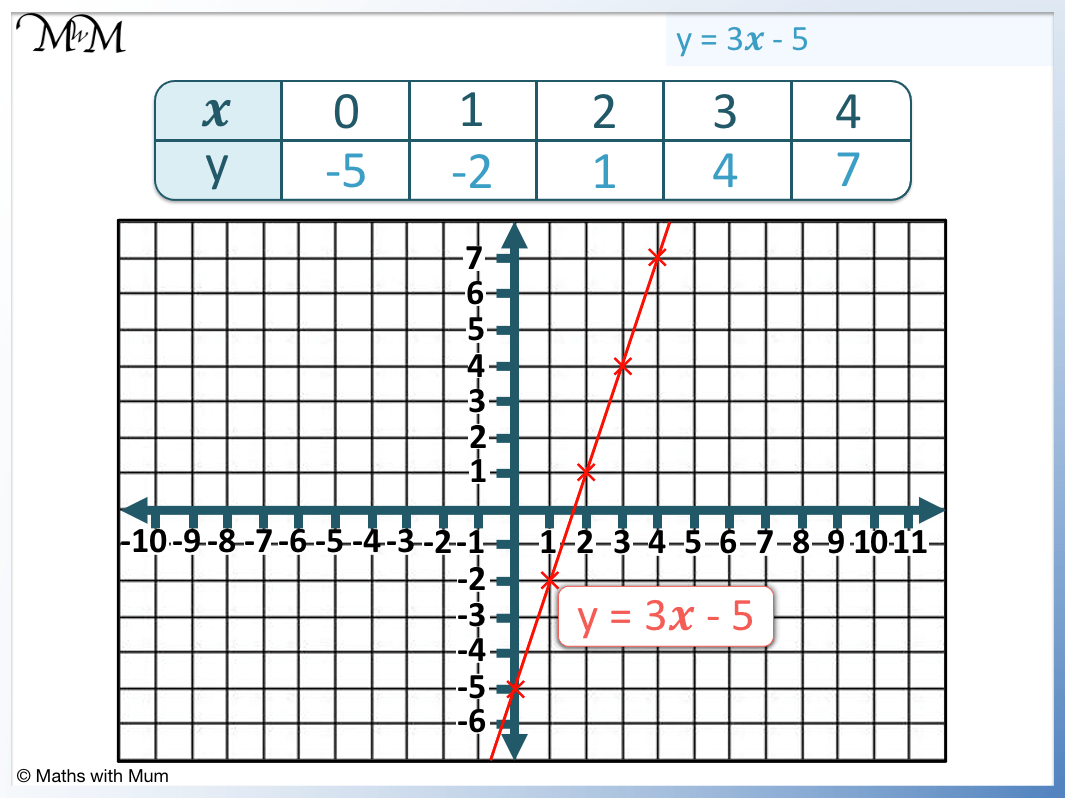• The table of values is created by substituting the given 𝑥 values into the equation y = 3𝑥 – 5.
• When 𝑥 = 0, y = 3𝑥 – 5 becomes y = -5. This forms the coordinates (0, -5).
• When 𝑥 = 1, y = 3𝑥 – 5 becomes y = -2. This forms the coordinates (1, -2).
• When 𝑥 = 2, y = 3𝑥 – 5 becomes y = 1. This forms the coordinates (2, 1).
• When 𝑥 = 3, y = 3𝑥 – 5 becomes y = 4. This forms the coordinates (3, 4).
• When 𝑥 = 4, y = 3𝑥 – 5 becomes y = 7. This forms the coordinates (4, 7).
• These coordinates are plotted and a line is drawn between the points.# Graphing a Line from a Table of Values

## What is a Table of Values?

A table of values contains two lists of numbers written alongside each other. The first list contains the chosen input values, which are often the 𝑥 coordinates. The second list contains the outputs obtained when this first list is put into a given equation, often the y coordinates. Together, the pairs of numbers in both lists make up coordinates that can be plotted as a graph.

In this example below, we have a table of values for y = 2𝑥 + 1.There are two lists of numbers shown in the table of values.

The first list is the given 𝑥 values. Here we chose 0 to 4. We can choose any numbers we like to go in the 𝑥 row. We chose 0 to 4 because they are easy to substitute into equations because they are small numbers.

The y values have been calculated using the equation y = 2𝑥 + 1. This equation tells us to multiply the 𝑥 values by 2 and then add one to them to find the y values.

For example, when × = 3, we multiply 3 by 2 and then add 1.

3 × 2 + 1 = 7 and so, we write 7 in the y row below the 𝑥 value of 3.

Doing this calculation for each 𝑥 value results in a different number which is written in the y row alongside the 𝑥 value it came from.

## How do you Make a Table of Values?

A table of values is made of two rows, the first labeled as 𝑥 and the second as y. In the 𝑥 row, consecutive numbers are chosen from between the smallest and largest 𝑥 coordinates on the axes given. The numbers in the y row are calculated by substituting these 𝑥 values into the given equation.

Here is a set of axes for plotting y = 2𝑥 – 3.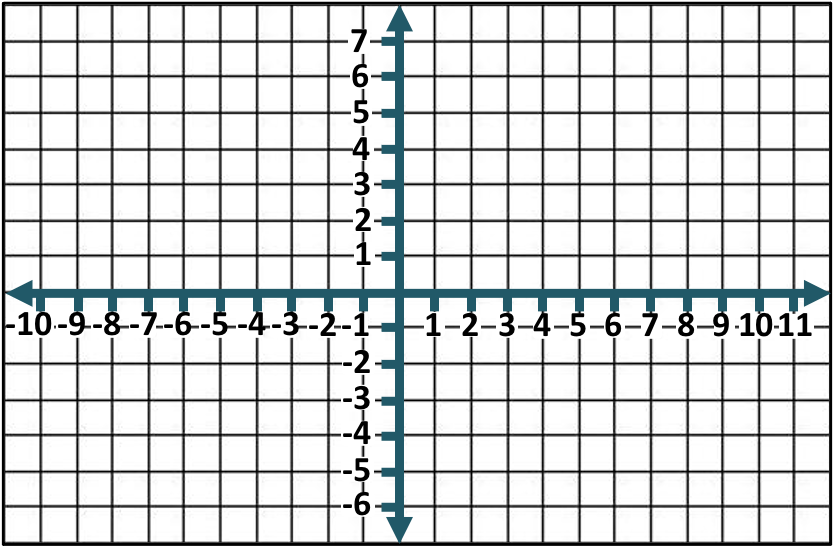The smallest 𝑥 value is -10 and the largest 𝑥 value is 10.

We only need two points to draw a straight line but it is a good idea to choose three to five different 𝑥 values for the table of values. This allows us to better notice any mistakes and to help us draw a more accurate line through the points.

To choose what 𝑥 values to use for the table of values, it is best to choose small positive whole numbers if possible.

In this example, we will choose 0, 1, 2, 3 and 4.The numbers in the y row are calculated by substituting these 𝑥 values into the equation y = 2𝑥 – 3. This tells us to multiply each 𝑥 value by 2 and then subtract 3.

The following table shows the calculations for the table of values.

𝑥 Coordinate Calculation for y = 2𝑥 – 3 y Coordinate
𝑥 = 0 y = 2 × 0 – 3 y = -3
𝑥 = 1 y = 2 × 1 – 3 y = -1
𝑥 = 2 y = 2 × 2 – 3 y = 1
𝑥 = 3 y = 2 × 3 – 3 y = 3
𝑥 = 4 y = 2 × 4 – 3 y = 5## How to Graph a Line from a Table of Values

A table of values contains pairs of 𝑥 and y values which form pairs of coordinates that can be plotted as points. The 𝑥 coordinate tells us how far right the point is and the y coordinate tells us how far up the point is. If the coordinates are negative, then the point is left or down respectively. Once each point is plotted, simply draw a line through them.

For example, here is the table of values for y = 3𝑥 – 5.We have the following coordinates:

• (0, -5) from 𝑥 = 0 and y = -5
• (0, -2) from 𝑥 = 1 and y = -2
• (0, 1) from 𝑥 = 2 and y = 1
• (0, 4) from 𝑥 = 3 and y = 4
• (0, 7) from 𝑥 = 4 and y = 7

The coordinates are plotted on the graph as shown.The following table explains how to plot coordinates:

Coordinate How to Plot it
Positive 𝑥 value Move to the right
Negative 𝑥 value Move to the left
Positive y value Move up
Negative y value Move down

• 𝑥 = 0 and y = -5 tells us to not go left or right and to go 5 down.
• 𝑥 = 1 and y = -2 tells us to go 1 right and 2 down.
• 𝑥 = 2 and y = 1 tells us to go right and 1 up.
• 𝑥 = 3 and y = 4 tells us to go 3 right and 4 up.
• 𝑥 = 4 and y = 7 tells us to go 4 right and 7 up

Each of these 2 numbers describe one point.

Once each point is plotted, the line is graphed by drawing a straight line through them all to the very edge of the axes.

## How to Tell if a Table of Values is Linear

A table of values is linear if as 𝑥 increases by a constant amount, the y values all increase by a constant amount. If the y values increase by the same amount from one number to the next, then the coordinates will form a straight line when plotted. If the y values increase by different amounts each time, then the table of values is non-linear.

If something is linear, this means that it forms a straight line.

For example, here is a table of values where the y values increase by 2 every time that 𝑥 increases by 1.Since the y values increase by 2 from one number to the next, this table of values is produced from a linear expression.

Alternatively, we can plot the 𝑥 and y values on a set of axes and look at it to see if it forms a straight line or not.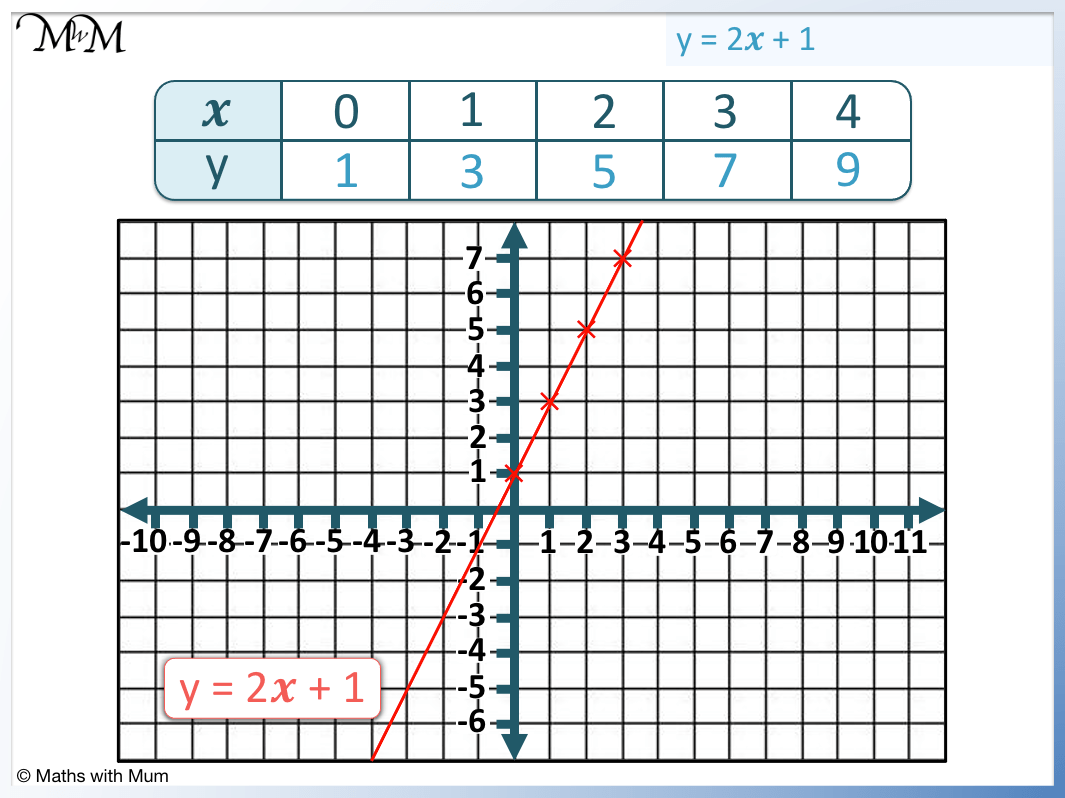We can see that the coordinates form a straight line and so, the table of values produces a linear graph.

If the equation that produced the table of values contains only y and 𝑥 as the variables, then it will produce a linear graph. If it contains 𝑥2 or any other powers of 𝑥, then it is non-linear.

This table of values is formed from the equation y= 2𝑥 + 1 and because there are no higher powers of 𝑥, the equation is a linear one.

## How to Find the Equation of a Straight Line From a Table of Values

The equation of a straight line is y = m𝑥 + c, where m is the gradient and c is the y-intercept. The gradient is the amount y increases every time that 𝑥 increases by 1 in the table. The y-intercept is the y value that accompanies the 𝑥 = 0 value in the table.

Here is an example of finding the equation from a table of values.The first step is to find the gradient. This is how much the y values increase every time that the 𝑥 values increase by 1.We can see that the y values increase by 3 every time the 𝑥 values increase by 1. The gradient is 3.

The second step is to find the y-intercept. This is the y value that is below the 𝑥 = 0 value. Below 𝑥 = 0 is y = -5. Therefore the y-intercept is -5.

The equation of the line is given by y = m𝑥 + c, where m is the gradient and c is the y-intercept.

The gradient of 3 is written in front of the 𝑥 and the y-intercept of -5 is just written afterward.

The equation of the line is y = 3𝑥 – 5.Now try our lesson on Right Angles where we learn how to identify right angles in a variety of situations.error: Content is protected !!